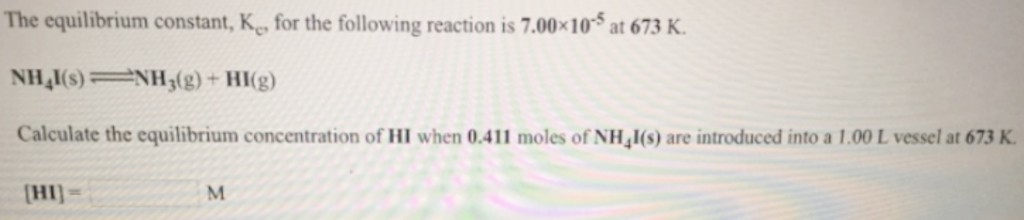# Problem: The equilibrium constant, Kc, for the following reaction is 7.00 x 10^-5 at 673 K.NH4I(s) NH3(g) + HI(g)Calculate the equilibrium concentration of HI when 0.411 moles of NH4I(s) are introduced into a 1.00 L vessel at 673 K.

###### FREE Expert Solution
80% (156 ratings)###### Problem Details

The equilibrium constant, Kc, for the following reaction is 7.00 x 10^-5 at 673 K.
NH4I(s) NH3(g) + HI(g)
Calculate the equilibrium concentration of HI when 0.411 moles of NH4I(s) are introduced into a 1.00 L vessel at 673 K.